# Basic Arithmetic : Subtraction with Whole Numbers

## Example Questions

### Example Question #1 : Subtraction With Whole Numbers

Paul had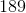video games. On Monday, he gavevideo games to his friend Chris. On Tuesday, he soldof his video games to the local video game shop. On Wednesday, Paul accidentally brokevideo games and threw them away. How many video games does Paul have left?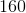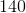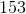Explanation:

This is a simple subtraction problem.

First, subtract the number of video games Paul gave to Chris on Monday.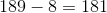Now, subtract the number of video games Paul sold from the number he has left.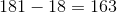Finally, subtract the number of video games Paul broke and threw away.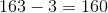### Example Question #13 : Operations With Whole Numbers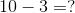What is the solution of the above equation?Explanation: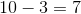We can show this using objects: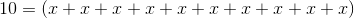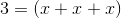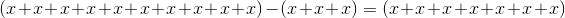We can see that only 7 objects are left.

### Example Question #12 : Operations With Whole Numbers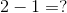What is the solution of the above equation?Explanation: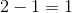We can see that by the objects.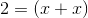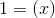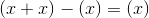We can see that there is only one object left.

### Example Question #11 : Whole Numbers

Timmy hassea monkeys.  At the end of the first month that he has them,sea monkeys die, and his parents buy himnew ones.  At the end of the second month,more sea monkeys die.  How many sea monkeys does Timmy have at the end of the second month?Explanation:

The problem can be solved as follows.  First, calculate the number of sea monkeys left at the end of the first month: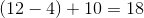Then, calculate the number of sea monkeys left at the end of the second month: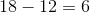### Example Question #2 : Subtraction With Whole Numbers

What is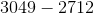?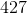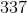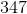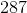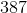Explanation: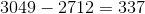If you need to break it down, you can split it into smaller pieces:and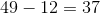, and then add the pieces back together: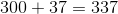.

### Example Question #14 : Operations With Whole Numbers

Solve for: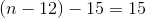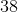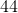Explanation:

To solve for n we need to isolate n on one side of the equation and all of the constants on the other side.We do this by adding 15 to both sides.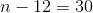Then we add 12 to both sides to get our final answer.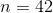### Example Question #2 : Subtraction With Whole Numbers

A city bus has five stops left on its route.  At the first stop,people get off.  At the second stop,get off.  At the third, no one gets off.  At the fourth,people get off.  At the fifth, there arepeople left on the bus, and they all get off.  How many people were on the bus before the first stop?Explanation:

To solve, create a linear equation:represents the total number of people on the bus before the first stop.

We then subtract the amount of people that got off at each stop and set that equal to the number of people to get off at the final stop.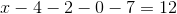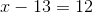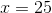### All Basic Arithmetic Resources